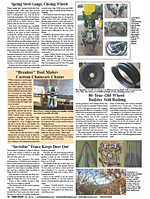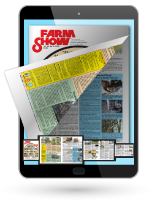"I've cut my electric bills drastically by determining which appliances and motors use too much electricity. Excess consumption often indicates a malfunction," says Jack Dwerlkotte, Marysville, Kan. He's figured out how to calculate his own electric bill by reading his electric meter, which he says is much more accurate than a conventional ammeter.
"It can tell you how many amps an individual motor draws, which tells you if it's overloaded for its horsepower rating. You can learn how much electricity a load of wash takes to dry in an electric dryer. You can determine how many cents per hour per day you're using and from that figure what your total bill will be at the end of the month if usage continues at the same rate," says Dwerlkotte.
"Look at the dial on your electric motor for the "Kh" symbol followed by a small number. Every time the meter wheel goes around, that's how many watts are consumed.
"With everything else shut off, my 5-hp. fan makes the meter go around 49 times a minute. My meter number reads Kh2 so, multiplying 49 times 2 equals 98 watts per min. To figure watts used per hour, multiply 98 times 60 and get 5,880 watts, or 5.88 kilowatts per hour. Multiply 5.88 times the 7.2 cent electric rate for this area and you get 42 cents per hour usage cost for that 5 hp. motor.
"To figure amperage drawn, divide 5.88 kilowatts by 230 volts to get 25.56 amps line draw. There are 746 watts to the horsepower so 5 hp. times 746 equals 3,730. You divide the number of watts consumed (5,880) by 3,730 and you get a 1.5764 service factor which may or may not indicate that the motor is overloaded.
"I often find that some motors use too much power. One way to test appliances like a deep freeze or refrigerator to see how much electricity they're consuming is to wire an electric clock into the unit and multiply the time it runs times the amount of current it draws using the method explained above."
Contact: FARM SHOW Followup, Jack Dwerlkotte, 604 N. 15, Marysville, Kan. 66508 (ph 913 562-2058).